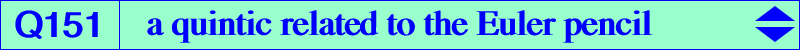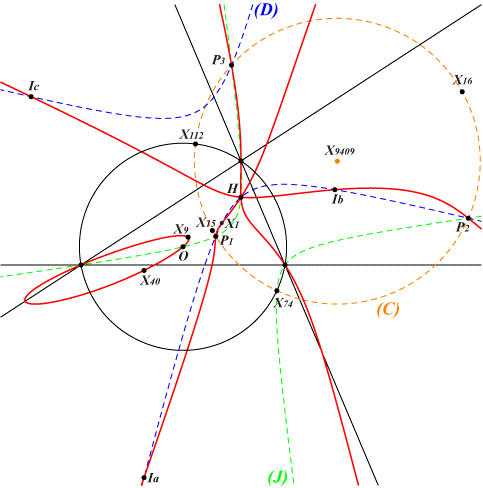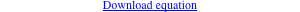too complicated to be written here. Click on the link to download a text file.X(1), X(3), X(4), X(9), X(40) excenters extraversions of X(9) and X(40)Table 27 contains the cubics of the Euler pencil. These are the isogonal pKs with pivot P on the Euler line. Any pK(X6, P) passes through the orthocenter H and the polar conic C(H) of H is a rectangular hyperbola passing through H and three other fixed points P1, P2, P3 studied in Table 27. When P traverses the Euler line, a pK(X6, P) meets its corresponding polar conic C(H) at six points (one being H itself counted twice) and then the locus of these points is the quintic Q151. See the related Q150. Properties • H is a triple point on Q151. • The tangents at A, B, C, X(3), the in/excenters all concur at H. • Q151 is invariant under the Cundy-Parry involution Ψ studied in CL037. • Q151 meets the Jerabek hyperbola (J) at A, B, C, X(3), X(4) counted three times and three other points P1, P2, P3. These points lie on the circle (C) with center X(9409), orthogonal to the circumcircle, which also contains X(74) on (J) and X(15), X(16), X(112). They also lie on the diagonal rectangular hyperbola (D) passing through the in/excenters and H.